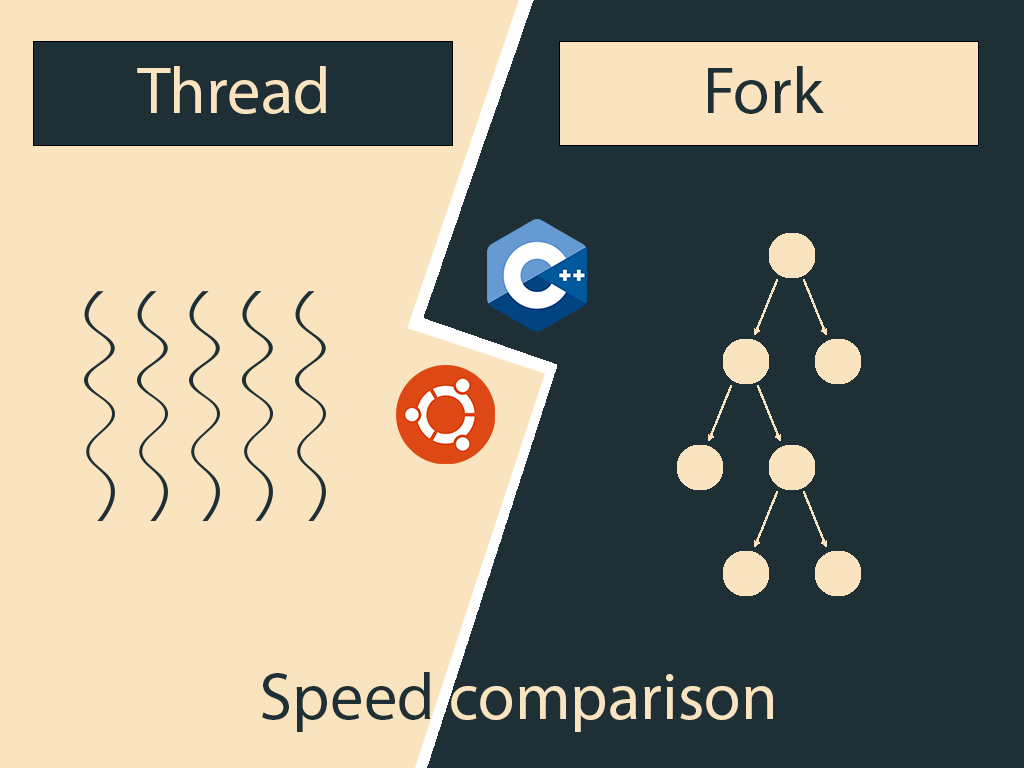# Threads vs forks in C++ - Speed test (Linux)

## Introduction

If you have a little experience in parallel programming, you certainly know the two approaches to code parallel applications : Threads (lightweight processes) and Forks (heavyweight processes).

In this article, we will see how matrix multiplication performs using those two approaches, and how to find the best number of threads or forks to create to make use of your whole CPU's power.

#### Notes :

• We will mainly talk about square matrices to simplify calculations.

• When I refer to operating system, I am actually talking about linux.

## Brief presentation of parallel programming

According to wikipedia, parallel programming (parallel computing) is a type of computation in which many processes are carried out simultaneously.

For instance, let's assume that we have a 100 * 100 matrix that we will call matA. We know that the result of matA * matA will be a 100 x 100 matrix. To perform this calculation, a naive approach would be to create as many processes as there are elements in the result matrix, i-e 100 * 100 = 10000 processes (the processes will execute "simultaneously").

The big questions of this article are : How efficient is this approach ? And what is the best approach ?

To answer those questions, let's start with some theory on how the CPU executes many processes (threads) simultaneously

## How does the CPU handle many threads

Whenever we execute a program in our computer, it runs on the CPU. But there is a lot of programs in our computers that are running at the same time. The operating system itself is a program (kernel). The desktop manager is a program. The terminal is a program. Every window you open is a program. But how does the CPU run them simultaneously ?

You've probably heard about CPU cores or multi-core processors. Let's go to wikipedia to see what it is :

A multi-core processor is a computer processor on a single integrated circuit with two or more separate processing units, called cores, each of which reads and executes program instructions.

But do we actually have as many cores as running programs in our computer ? The answer is NO. The most powerful public computers today (2021) are usually limited at 8 cores. Does that mean that we can only run 8 programs at the same time ?

Fortunately, the answer is no. The operating system (more specifically the kernel), has what is called A scheduler. To simulate a parallel behaviour in our computer, the scheduler is in charge of context switching. For instance, if we have more than 8 programs running in an 8 cores CPU, the scheduler gives each program a bit of time in which it can perform a part of its task, and then pauses it to let another program run for a bit of time, and so on. This is the lifecycle of processes in linux :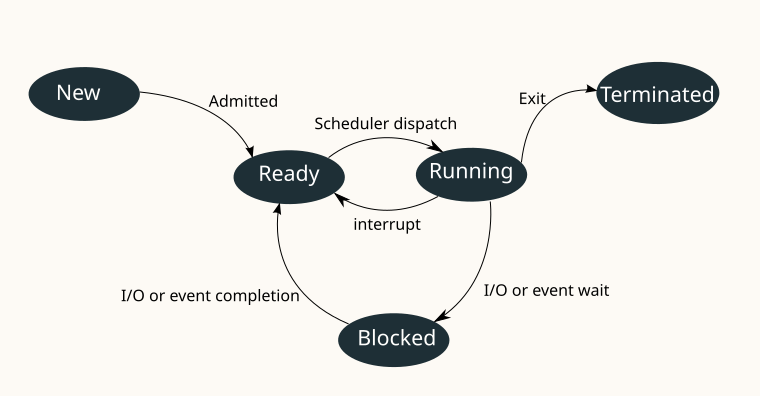Program lifecycle states in linux (source)

The most important part for us is the one between Ready and Running states, because it's where the scheduler operates.

## Threads & Forks

We talked early in this article about parallel programs. Threads and Forks are two ways to create them. They are similar in the way the CPU sees them (in linux) (sched man page), but are different from a memory management perspective.

In memory, a process is devided into 3 segments :

• Code segment

• Data segment

• Stack and queue segment

On the one hand, when performing a fork() call, the parent process (the caller) duplicates to create a child process. After a fork() call, there are two processes with different PIDs. The system actually creates a copy of all the segments of the parent process, something that is pretty heavy. This is why forked processes are also called "heavyweight processes".

On the other hand, creating a thread does not duplicate segments. It only creates a context (see Thread control block for more informations) that stores the state of progress of the newly created thread : its program counter, its stack pointer, ... etc. This gives the thread access to the data (data segment) of the parent process as it is not duplicated, while being able to run simultaneously with the main thread.

## Performance comparison

To compare performance between threads and forks, I made a program that performs a matrix multiplication. You can check out the code here.

### Fork code :

As I said above, when performing a fork(), the segments are duplicated. The two processes (parent and child) cannot see the modifications of each other. At first sight, this is problematic for us because we need to perform a matrix multiplication and thus store the data at a unique emplacement.

Fortunatly, unix operating system offers us the possibility to create an inter-process shared space. There are many mechanisms, but I chose mmap(). See the MAP_SHARED for shared memory space creation. Here is my constructor of a matrix :


Matrix::Matrix(uint lines, uint columns, bool shared) {
_shared = shared;
if (shared) {
// Creating the shared memory space for the container of
// lines (table of tables)
_elementsMatrix = (float**)mmap(
NULL,
sizeof(float*) * lines,
MAP_SHARED | MAP_ANONYMOUS,
-1,
0
);

// Creating shared memory space for lines
for (int i = 0; i < lines; ++i) {
_elementsMatrix[i] = (float*)mmap(
NULL,
sizeof(float) * columns,
MAP_SHARED | MAP_ANONYMOUS,
-1,
0
);
// Initializing the line with 0's
float elements[columns] = {0};
memcpy(_elementsMatrix[i], &elements, columns * sizeof(float));
}

} else {
_elementsMatrix = new float*[lines];
for (int i = 0; i < lines; ++i) {
_elementsMatrix[i] = new float[columns];
}
}

// Initializing lines and columns
_lines = lines;
_columns = columns;

}



And here is the code to create the processes using fork() (checkout my GitHub for the complete code) :


void Matrix::multiplyUsingForks(
const int & nbThreads,
const Matrix & matrix1,
const Matrix & matrix2,
Matrix * solution
) {

// Get the number of elements for each thread
unsigned int matrix1Lines = matrix1._lines;
unsigned int matrix1Columns = matrix1._columns;
unsigned int matrix2Lines = matrix2._lines;
unsigned int matrix2Columns = matrix1._lines;

unsigned int nbElements = matrix1Lines * matrix2Columns;

pid_t pid;

// security
if (nbThreads > nbElements) {
}

// Get the interval of values to calculate by each process
for (int i = 0; i < _nbThreads; ++i) {
thresholds[i].lower = i * elementsPerThread;
thresholds[i].upper = (i + 1) * elementsPerThread - 1;
}
thresholds[_nbThreads - 1].upper = nbElements - 1;

// Creating the processes
for (int i = 0; i < _nbThreads; ++i) {
pid = fork();
if (pid == 0) {
multiplyElements(
matrix1,
matrix2,
solution,
thresholds[i]
);
exit(0);
}
}

// Synchronization
if (pid != 0) {
while (wait(NULL) > 0);
}

}



Unlike forks, threads are less expensive to create. The system juste creates a context (Thread control block) that shares the data segment with the creator thread. Thus, there is no need to use an inter-process memory sharing mechanism.

Here is the code I used to create threads :


const Matrix & mat1,
const Matrix & mat2,
Matrix * resultMatrix,
const limits & thresholds
) {

Matrix::multiplyElements(
mat1,
mat2,
resultMatrix,
thresholds
);

// Notify the main thread to check if all the threads have finished the job
{
lock_guard lock(resultMatrix->_mt);
--resultMatrix->_nbProcesses;
resultMatrix->cv.notify_one();
}

}

const unsigned int & nbThreads,
const Matrix & matrix1,
const Matrix & matrix2,
Matrix * solution
) {
// Get the number of elements for each thread
unsigned int matrix1Lines = matrix1._lines;
unsigned int matrix1Columns = matrix1._columns;
unsigned int matrix2Lines = matrix2._lines;
unsigned int matrix2Columns = matrix1._lines;

unsigned int nbElements = matrix1Lines * matrix2Columns;

// security
if (nbThreads > nbElements) {
}

for (int i = 0; i < _nbThreads; ++i) {
thresholds[i].lower = i * elementsPerThread;
thresholds[i].upper = (i + 1) * elementsPerThread - 1;
}
thresholds[_nbThreads - 1].upper = nbElements - 1;

for (int i = 0; i < _nbThreads; ++i) {
ref(matrix1),
ref(matrix2),
solution,
ref(thresholds[i])
);
}

// Barrier synchronization
{
unique_lock < mutex > lock (solution->_mt);
solution->cv.wait(lock, [&solution] {return solution->_nbProcesses == 0;});
}

// No need to join detached threads, just free the resources
for (int i = 0; i < _nbThreads; ++i) {
}

}



Now, let's take a look at performances of each approach.

### Specifications :

As benchmark results are hardware specific, here is the lscpu output of my benchmarking environment :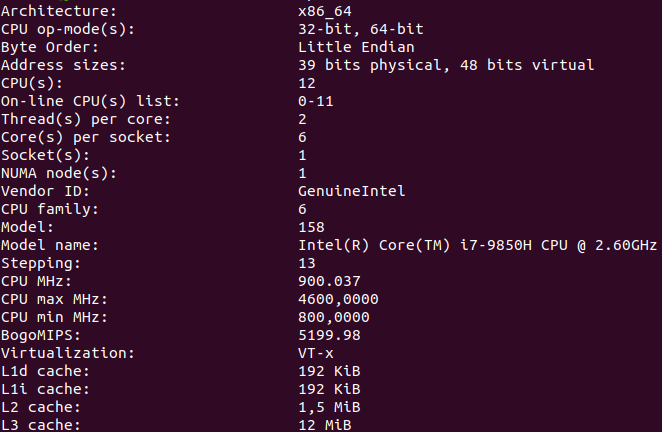lscpu output

The results shown below are the made up from an average of 10 consecutive runs

## Benchmark results :

Theoretically, as we said that threads are more lightweight than forks, we can expect better performances for threads. Let's see :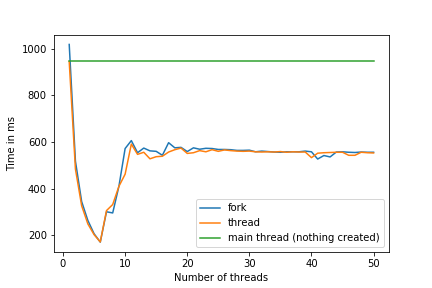Threads vs forks performances

Now we can see that threads are really slightly better, but why isn't there a big difference ?

### Copy on write mechanism :

Fortunately, to speed up forks, linux has foreseen this kind of situation and provided the copy on write mechanism to efficiently duplicate processes.

When duplicating a process, instead of immediately duplicating all the 3 segments we talked about above, the two processes will actually share the data as long as no one modifies it. With this, the data is then copied only when one of the processes makes modifications on it. This will allow the system to speed up process duplicating, but it slows down the first modification (it there is one).

In our program, the only write I performed on the shared data is the one into the "result matrix". Because it is actually located in an inter-processes shared space (see mmap() above), there is no copy and therefore no performance loss.

### The use of atomics :

In order to synchronize all the threads, I used an atomic integer, which offers inter thread synchronization.

First, I declared this variable, which is a member of Matrix class :


/**
* @brief Number of active processes that are accessing the matrix
*
* @note It is used to synchronize processes
*
*/
atomic< int > _nbProcesses;



Then, I used it for synchronization by initializing it to the number of threads that will be created, and decrementing it whenever a thread is done. With this said, If I want to know if all the threads are done, I only check if the value of this atomic variable is equal to 0 :





// Notify the main thread to check if all the threads have finished the job
{
lock_guard lock(resultMatrix->_mt);
--resultMatrix->_nbProcesses;
resultMatrix->cv.notify_one();
}



Atomics are a simple way to get an inter threads synchronization, but in return, we get a little drop in performance. Refer to this YouTube video to see this in details (See conditional variables in C++ for more details on the code).

So, atomics are a small disadvantage for threads in our example.

## Why is execution time getting worse when creating a lot of threads

In our graph, we can see that performances are getting worse when we create more than 6 threads. This is because of the context switch we talked about above in this article.

In our computers, we usually have multicore CPUs. Mine is 6 cores. This means that it can execute 6 processes (threads) at the same time, but in reality, I can run much more programs simultaneously. This is done by the context switch we talked about earlier in this article. But how does context switch work ?

To process a program, the CPU has a bench of registers (Program counter, variables registers, stack pointers, ... etc). When switching the context, the content of all those registers is put into memory (Actually there is more data that is stored and restored. See context switch for more information).

Let's take an example to illustrate what happens when we have 3 programs (A, B & C) in a single core CPU :

• Program A runs for 10 ms

• The CPU stores the state registers of the program A in memory

• The CPU loads the state of the program B from memory

• Program B runs for 10 ms

• The CPU saves the state registers of the program B into memory

• The CPU loads the state of the program C from memory

• Program C runs for 10 ms

• ...

This is just an example of First come first served strategy (see this wikipedia article for more information) but it illustrates the memory accesses we have when having more processes than CPU cores. Since those are expensive in terms of access time, creating more processes than CPU cores is a disadvantage.

## What is the best number of threads to create ?

As we have seen in this benchmark results, the best execution times we obtained was when using 6 threads (or processes), which is exactly the number of cores I have on my CPU :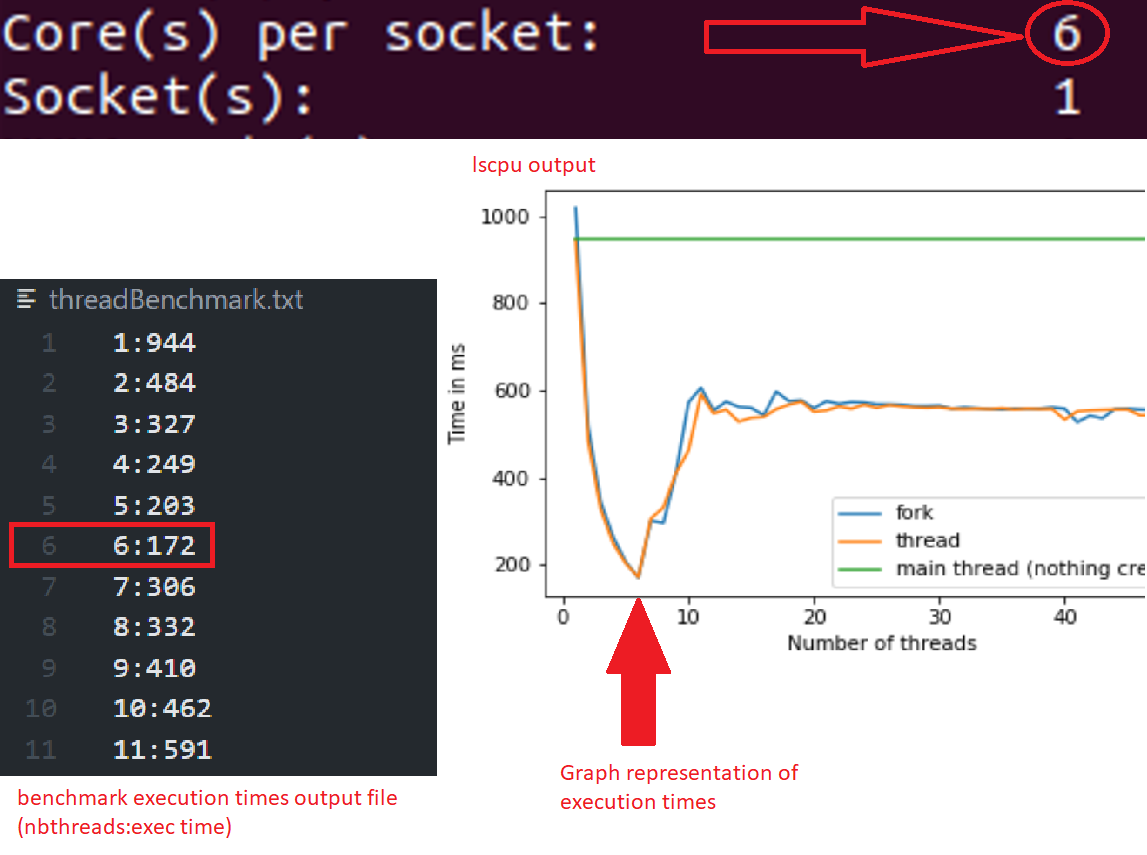benchmark run in a 6 core CPU

I also run the benchmark in a 4 cores CPU, here is the result :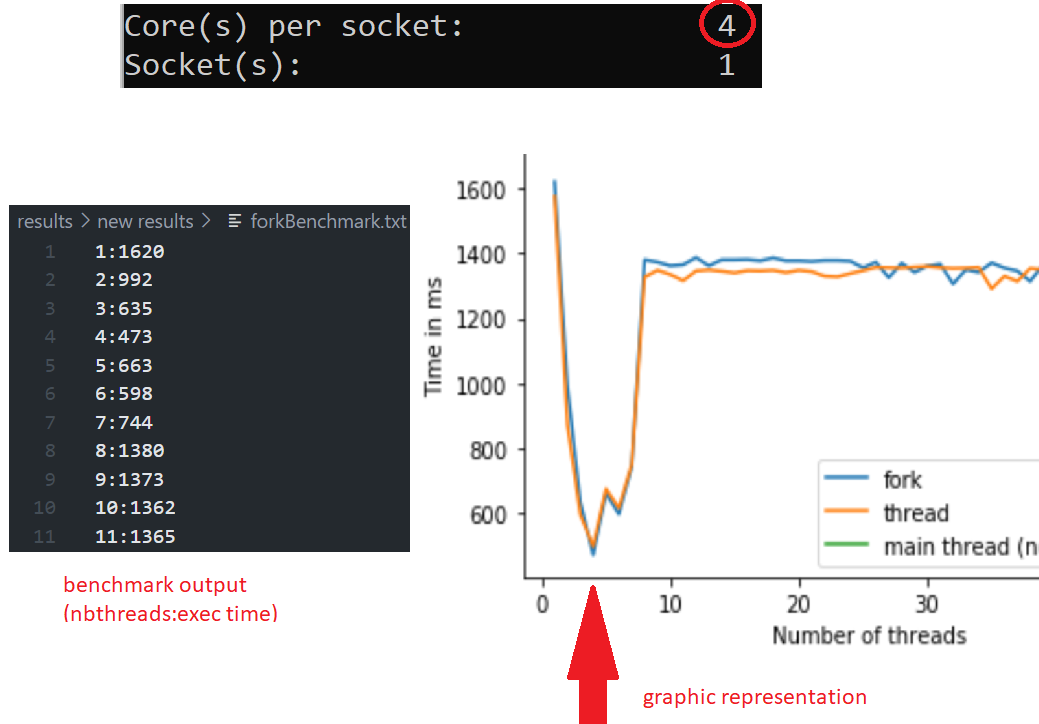benchmark run in a 4 core CPU

Thus, we can conclude that the optimal number of threads to create depending on the hardware is the number of CPU cores. If you have a 6 core CPU, then create 6 threads/forks. If you have 4 core CPU, then create 4 threads/forks, and so on.

Please feel free to report any problem you find in the article by reaching me on instagram